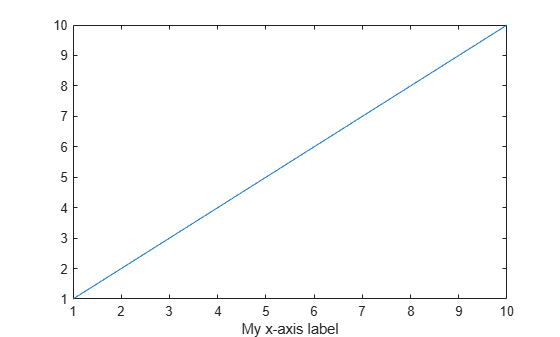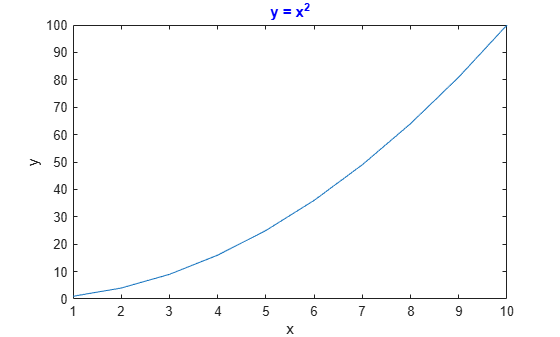Main Content

# findall

Find all graphics objects

## Syntax

``h = findall(objhandles)``
``h = findall(objhandles,prop1,value1,...,propN,valueN)``

## Description

example

````h = findall(objhandles)` returns the graphics objects in `objhandles` and all of their descendants. Unlike the `findobj` function, `findall` returns objects even if their `HandleVisibility` property is set to `'off'`.```

example

````h = findall(objhandles,prop1,value1,...,propN,valueN)` returns the handles of all objects in the hierarchy that have the specified properties set to the specified values. For example, ```h = findall(gcf,'Type','text',Color','r')``` returns all text objects in the current figure that have a red color.```

## Examples

collapse all

Create three figures. Set the `HandleVisibility` property of the last figure to `'off'`.

```f1 = figure; f2 = figure; f3 = figure('HandleVisibility','off');```

Display the number of visible or hidden handles in the graphics object hierarchy. Your result might be different from the result shown.

```h1 = findall(groot); disp(numel(h1))```
``` 4 ```

Find all visible or hidden figures.

`h2 = findall(groot,'Type','figure')`
```h2 = 3x1 Figure array: Figure (3) Figure (2) Figure (1) ```

If you try to find the figures using the `findobj` function, MATLAB® returns only `f1` and `f2`.

`h3 = findobj('Type','figure')`
```h3 = 2x1 Figure array: Figure (2) Figure (1) ```

`Text` objects within a figure have hidden handles. Use `findall` to return these hidden handles.

Create a figure with a plot. Then, create a label for the x-axis.

```plot(1:10) txt = xlabel('My x-axis label');```Verify that the `HandleVisibility` property on `txt` is set to `'off'`.

`txt.HandleVisibility`
```ans = 'off' ```

Use `findall` to return the `Text` object for the x-axis label.

`h1 = findall(gcf,'Type','text')`
```h1 = Text (My x-axis label) with properties: String: 'My x-axis label' FontSize: 11 FontWeight: 'normal' FontName: 'Helvetica' Color: [0.1500 0.1500 0.1500] HorizontalAlignment: 'center' Position: [5.0000 0.3947 -1] Units: 'data' Show all properties ```

Because the `Text` object is hidden, you cannot find it using the `findobj` function.

`h2 = findobj(gcf,'Type','text')`
```h2 = 0x0 empty GraphicsPlaceholder array. ```

Use `findall` to return all `Text` objects or `Text` objects with specific properties.

Create a figure with a plot. Then, label the axes and add a title to the axes. Set the color of the title to blue.

```plot((1:10).^2) xlabel('x') ylabel('y') title('y = x^2','Color','b')```Return all `Text` objects in the current figure.

`h1 = findall(gcf,'Type','text')`
```h1 = 3x1 Text array: Text (y = x^2) Text (x) Text (y) ```

Now, return all blue `Text` objects.

`h2 = findall(gcf,'Type','text','Color','b')`
```h2 = Text (y = x^2) with properties: String: 'y = x^2' FontSize: 11 FontWeight: 'bold' FontName: 'Helvetica' Color: [0 0 1] HorizontalAlignment: 'center' Position: [5.0000 100.7725 1.4211e-14] Units: 'data' Show all properties ```

## Input Arguments

collapse all

Objects to search from, specified as an array of graphics objects. `findall` searches the objects in the input array `objhandles` and all of their descendants in the graphics object hierarchy.

Example: `h = findall(groot)` returns all visible and hidden handles in the graphics object hierarchy.

Property name, specified as a character vector or string scalar. For more information, see Graphics Object Properties.

Example: `h = findall(gcf,'Type','text')` returns all objects in the current figure whose `Type` property is set to `'text'`.

Property value, specified as a scalar or array.

## Tips

• To customize your search with `findall`, you can use `objhandles` followed by the input combinations from the `findobj` function. For example:

```h = findall(groot,prop1,value1,'-not',prop2,value2,'-property',prop3) ```

## See Also

### Topics

Introduced before R2006a

Download ebook